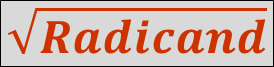Original Puzzle...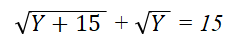rearranging...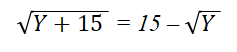squaring both members...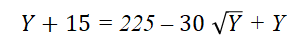rearranging...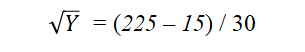performing arithmetic...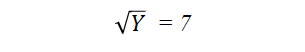squaring both members...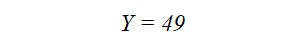most influential book in the thought-life of your puzzle-master is a small volume entitled How to Solve It by Hungarian mathematician George Pólya.  The work is framed on four principles

1.     Understand the problem (puzzle).

2.     Devise a plan.

3.     Carry out the plan.

4.     Review / Extend.

The most important – and creative! – is signified by the word “Extend,” which I have colloquialized for my students: “Here is a solution to a problem what other problems can I now solve.”  That will be seen as the heuristic concept selected for emphasis in Discovering Assumptions.

Let us apply the fourth principle by generalizing the problem/puzzle with the parameter X as a replacement for the fixed integer 15...

Original Puzzle...parametric replacement...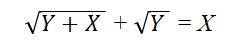rearranging...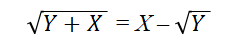squaring both members...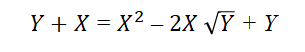rearranging...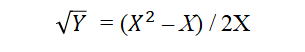algebraic simplification...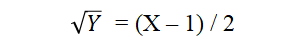squaring both members...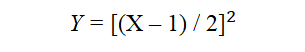...which produces an infinite number of solutions as shown in this graph...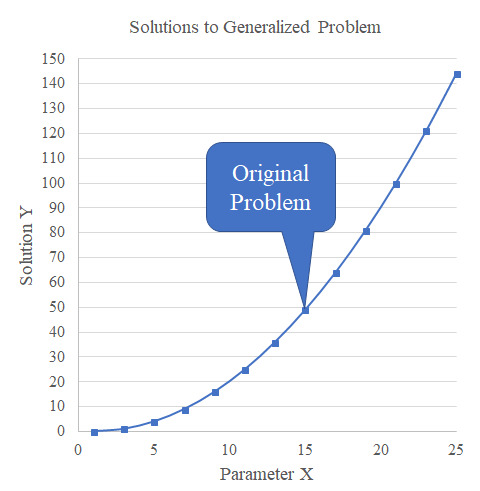We will also review our generalized solution by substituting X = 15, et voilà: Y = 49.

Bonus Puzzle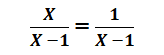Simple: Just multiply both members of the equation by the expression X – 1, et voilà: X = 1.  Here again we will review our solution by substituting X = 1 into the original equation, et voilà: 1 / 0 = 1 / 0.  Oh, but wait: 1 / 0 = ......which reminds solvers of the mischief revealed in Double Integrity.

Epilog

Recommendation from John Swanson:  Graph of x / (x-1) in blue and 1 / (x-1) in red...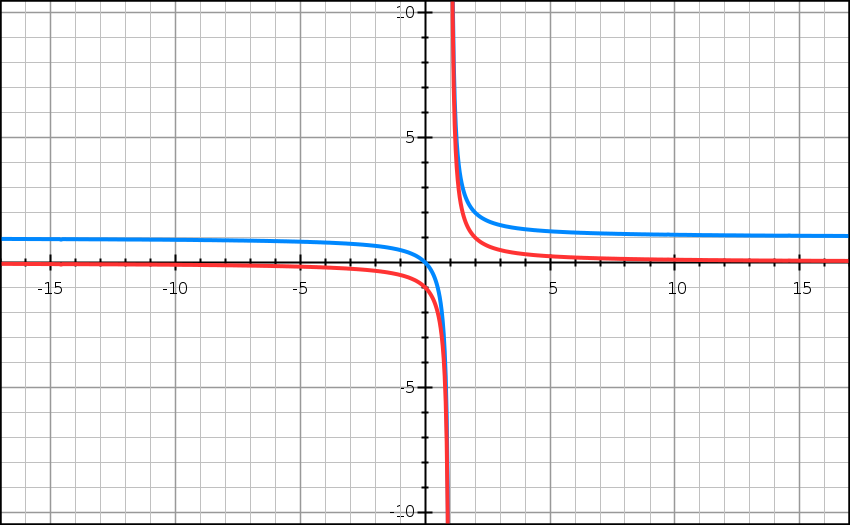Home Page Puzzle Page Math and Models The Puzzle as a Literary Genre